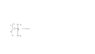# Force of a charge Q at the origin?

• Violagirl

## Homework Statement

A charge Q is placed at the center of the origin on the attached document. What is the force on this charge?

Coulomb's Law:

F= kqQ/d2 ^
r

## The Attempt at a Solution

See attached document for figure. I'm confused by this problem. Along the positive y axis, we have a postive charge of Q. On the negative y axis, we have 2Q, which I also assume is a positive charge. Hence because Q at the origin is also at the center, I thought that both charges would repel this Q to stay at the center because like charges repel each other. I'm not sure how to show this though with Coloumb's Law since from that point of view, Q isn't going anywhere so there would be no change in distance...

#### Attachments

•Force on Q at origin.jpg
3.1 KB · Views: 766

I'm confused by this problem. Along the positive y axis, we have a postive charge of Q. On the negative y axis, we have 2Q, which I also assume is a positive charge. Hence because Q at the origin is also at the center, I thought that both charges would repel this Q to stay at the center because like charges repel each other. I'm not sure how to show this though with Coloumb's Law since from that point of view, Q isn't going anywhere so there would be no change in distance...
In your expression for Coulombs law, you forgot that force is a vector.
How would you normally handle two forces that point in opposite directions?

Well, thinking of it as a vector, we have a force from Q at origin to Q going upward. And then there's a second force from Q at origin to 2Q for where 2Q has a vector pointing downward. So would I find the difference between the vectors of both charges and use Coloumb's Law twice in the problem for both situations and then subtract the equations for both?

Well done.
Just some pointers...

It's hard to talk about isn't it. It can help to rewrite the question in more precise terms...
Lets set normal cartesian x-y axis, then fixed charges p=+Q and r=+2Q at at position (0,b) and (0,-2b) respectively.
You want to know the net force on charge q=+Q at position (0,0).
You can draw a free-body diagram for charge q
... the force on q due to r is Fr, and it points which way?
... the force on q due to p is Fp, and it points which way?

The net force in the +y direction is given by... F(net)=...

Interestingly you don't need to apply the Coulombs law equation and the algebra etc to see what will happen.
You can use what you know of the consequences...

twice the charge= how many times the force?
but: twice the distance = how many times the force?
... so: if you double the charge and also double the distance, how many times the force?
... so Fr is how many times Fp?

Last edited:
Thank you so much for your input! If you increase distance between two charged points, you will greatly reduce the impact of the force. Increasing the charge with an increase in distance will still reduce how much force is generated between both points.

"greatly reduce"? How many times? Can you put a number on that?
This is actually the core lesson yu are supposed to learn from the exercize.

Note: you can still increase the charge and the distance and still have increased force.
("impact of force" and "force" are the same thing - but I think you mean "magnitude of force".)